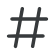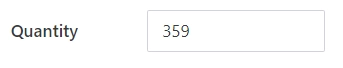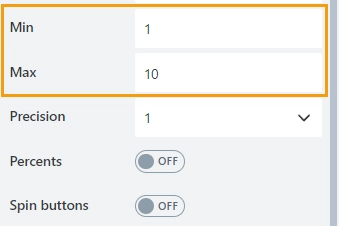#Number

Allows a user to enter a numeric value. The field value can be displayed as a number, currency, or percentage.

This page contains a detailed description of the field properties and JavaScript samples which you can use with this field.General

Title

Control

## Number Properties

Here you can find properties specifically related to the Number field.

### Min/Max

Specify the minimum and maximum values that a user can enter:When a user enters a value outside the range, the value changes to the nearest valid value:### Decimals

Specify the number of decimal places to which the number will be rounded. The default property value is 0.### Format

Specify the number format that is applied when a user leaves the control. The default property value is n0.The number format is made up of format specifier and the number of decimals places. E.g. n3, c2.

Available format specifiers:

• ‘n’ — Renders a number.

• ‘c’ — Renders a currency value.

• ‘p’ — Renders a percentage (number is multiplied by 100).

• ‘e’ - Renders exponential values.

### Step

Specify the interval for adjusting the current value when using up and down arrows:You can enter either an integer or a decimal number.

## JavaScript framework

In this section, you can find basic examples of how to work with the field using JavaScript.

For more examples, check out Working with form fields in JavaScript article. If you are not familiar with the JavaScript framework, get started with the JavaScript basics.

Note

The field is only accessible once the form is rendered, so all calls to the field must be inside fd.rendered event:

```fd.rendered(function(){
//make field required
fd.field('Field1').required = true;
});
```

### Get or set field value

Get or set the Number field value:

```//return field value
fd.field('Field1').value;

//set field value
fd.field('Field1').value = 152;
```

### Handle change event

Execute a function when a field value has been changed:

```fd.field('Field1').\$on('change', function(value) {
//log changes to browser's console
console.log('New value: ' + value);
});
```

### Make field required

Make a field required or optional:

```//make field required
fd.field('Field1').required = true;

//make field not required
fd.field('Field1').required = false;
```

### Disable field

Make a field non-editable. The field value can still be changed with JavaScript and saved:

```//disabled field
fd.field('Field1').disabled = true;

//enable field
fd.field('Field1').disabled = false;
```

### Get HTML element

Access HTML element inside the field in order to modify it, hide it, or do something else.

```//access field's control
var htmlField = fd.field('Field1').\$el;

//access field's block, which includes title and control
var htmlFullField = fd.field('Field1').\$parent.\$el;
```

### Hide field

Hide a field from a user. The field value can still be accessed and changed with JavaScript:

```//hide field
fd.field('Field1').hidden = true;

//show field
fd.field('Field1').hidden = false;
```

### Cоnfigure widget

You can access the widget used by the field. The widget is based on Kendo UI NumericTextBox.

```// get the widget
fd.field('Field1').widget

// change the widget's configuration
fd.field('Field1').widgetOptions = {
spinners: false
}
```

widgetOptions is the same as widget.setOptions({}) but can be defined before widget initialization.

### Number of decimal places

Specify the number of decimal places to which the number will be rounded:

```fd.field('Field1').widgetOptions = {
decimals: 3
}
```

### Displayed number format

Specify the number format that is applied when a user leaves the control.

The number format is made up of format specifier and the number of decimals places. E.g. n3, c2.

Avaliable format specifiers:

• ‘n’ — Renders a number.

• ‘c’ — Renders a currency value.

• ‘p’ — Renders a percentage (number is multiplied by 100).

• ‘e’ - Renders exponential values.

```fd.field('Field1').widgetOptions = {
format: "n2"
}
```

### Limit the range of number

Specify the minimum and maximum values that a user can enter:

```fd.field('Field1').widgetOptions = {
min: 5,
max: 99
}
```

### Restrict decimals

Specify whether the decimals length should be restricted during typing. The number of decimal places is defined by the decimals property value.

```fd.field('Field1').widgetOptions = {
decimals: 3,
restrictDecimals: true
}
```

### Rounded

Specify whether the value should be rounded or truncated. The default property value is true.

```fd.field('Field1').widgetOptions = {
round: false
}
```

### Step

Specify the interval for adjusting the current value when using up and down arrows. The default property value is 1.

```fd.field('Field1').widgetOptions = {
step: 0.01
}
```